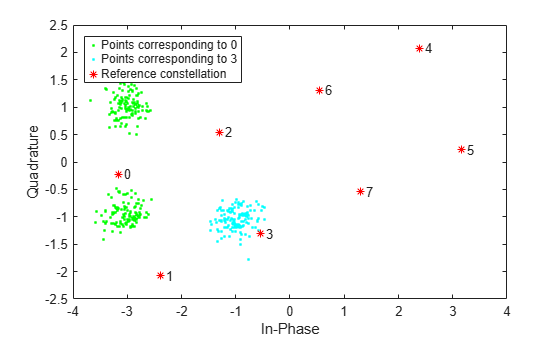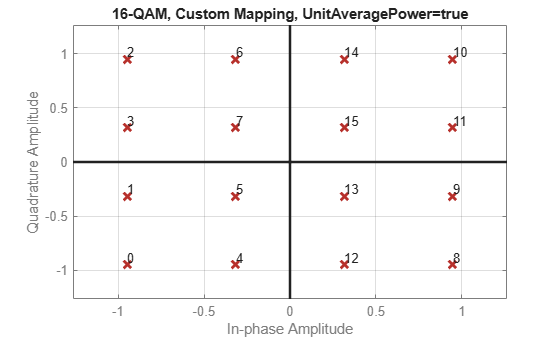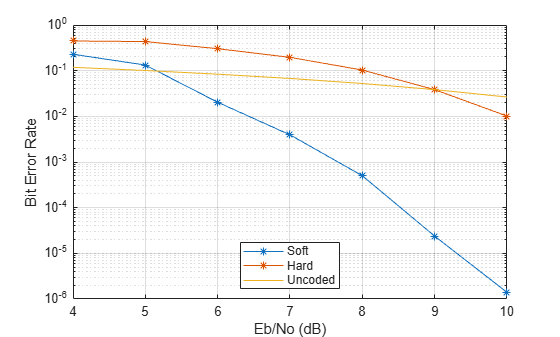# qamdemod

## Syntax

``z = qamdemod(y,M)``
``z = qamdemod(y,M,symOrder)``
``z = qamdemod(___,Name,Value)``

## Description

example

````z = qamdemod(y,M)` returns a demodulated signal, `z`, given quadrature amplitude modulation (QAM) signal `y` of modulation order `M`.```

example

````z = qamdemod(y,M,symOrder)` returns a demodulated signal, `z`, and specifies the symbol order for the demodulation.```

example

````z = qamdemod(___,Name,Value)` specifies options using one or more name-value pair arguments. For example, `'OutputType','bit'` sets the type of output signal to bits.```

## Examples

collapse all

Demodulate an 8-QAM signal and plot the points corresponding to symbols 0 and 3.

Generate random 8-ary data symbols.

`data = randi([0 7],1000,1);`

Modulate `data` by applying 8-QAM.

`txSig = qammod(data,8);`

Pass the modulated signal through an AWGN channel.

`rxSig = awgn(txSig,18,'measured');`

Demodulate the received signal using an initial phase of $\pi$/8.

`rxData = qamdemod(rxSig.*exp(-1i*pi/8),8);`

Generate the reference constellation points.

`refpts = qammod((0:7)',8) .* exp(1i*pi/8);`

Plot the received signal points corresponding to symbols 0 and 3 and overlay the reference constellation. The received data corresponding to those symbols is displayed.

```plot(rxSig(rxData==0),'g.'); hold on plot(rxSig(rxData==3),'c.'); plot(refpts,'r*') text(real(refpts)+0.1,imag(refpts),num2str((0:7)')) xlabel('In-Phase') ylabel('Quadrature') legend('Points corresponding to 0','Points corresponding to 3', ... 'Reference constellation','location','nw');```Modulate and demodulate random data by using 16-QAM with WLAN symbol mapping. Verify that the input data symbols match the demodulated symbols.

Generate a 3-D array of random symbols.

`x = randi([0,15],20,4,2);`

Create a custom symbol mapping for the 16-QAM constellation based on WLAN standards.

`wlanSymMap = [2 3 1 0 6 7 5 4 14 15 13 12 10 11 9 8];`

Modulate the data, and set the constellation to have unit average signal power. Plot the constellation.

`y = qammod(x,16,wlanSymMap,'UnitAveragePower', true,'PlotConstellation',true);``z = qamdemod(y,16,wlanSymMap,'UnitAveragePower',true);`

Verify that the demodulated signal is equal to the original data.

`isequal(x,z)`
```ans = logical 1 ```

Demodulate a fixed-point QAM signal and verify that the data is recovered correctly.

Set the modulation order as `64`, and determine the number of bits per symbol.

```M = 64; bitsPerSym = log2(M);```

Generate random bits. When operating in bit mode, the length of the input data must be an integer multiple of the number of bits per symbol.

`x = randi([0 1],10*bitsPerSym,1);`

Modulate the input data using a binary symbol mapping. Set the modulator to output fixed-point data. The numeric data type is signed with a 16-bit word length and a 10-bit fraction length.

```y = qammod(x,M,'bin','InputType','bit','OutputDataType', ... numerictype(1,16,10));```

Demodulate the 64-QAM signal. Verify that the demodulated data matches the input data.

```z = qamdemod(y,M,'bin','OutputType','bit'); s = isequal(x,double(z))```
```s = logical 1 ```

Estimate bit error rate (BER) performance for hard-decision and soft-decision Viterbi decoders in AWGN. Compare the performance to that of an uncoded 64-QAM link.

Set the simulation parameters.

```clear; close all rng default M = 64; % Modulation order k = log2(M); % Bits per symbol EbNoVec = (4:10)'; % Eb/No values (dB) numSymPerFrame = 1000; % Number of QAM symbols per frame```

Initialize the BER results vectors.

```berEstSoft = zeros(size(EbNoVec)); berEstHard = zeros(size(EbNoVec));```

Set the trellis structure and traceback depth for a rate `1/2`, constraint length `7`, convolutional code.

```trellis = poly2trellis(7,[171 133]); tbl = 32; rate = 1/2;```

The main processing loops performs these steps:

• Generate binary data

• Convolutionally encode the data

• Apply QAM modulation to the data symbols. Specify unit average power for the transmitted signal

• Pass the modulated signal through an AWGN channel

• Demodulate the received signal using hard decision and approximate LLR methods. Specify unit average power for the received signal

• Viterbi decode the signals using hard and unquantized methods

• Calculate the number of bit errors

The `while` loop continues to process data until either 100 errors are encountered or ${10}^{7}$ bits are transmitted.

```for n = 1:length(EbNoVec) % Convert Eb/No to SNR snrdB = EbNoVec(n) + 10*log10(k*rate); % Noise variance calculation for unity average signal power. noiseVar = 10.^(-snrdB/10); % Reset the error and bit counters [numErrsSoft,numErrsHard,numBits] = deal(0); while numErrsSoft < 100 && numBits < 1e7 % Generate binary data and convert to symbols dataIn = randi([0 1],numSymPerFrame*k,1); % Convolutionally encode the data dataEnc = convenc(dataIn,trellis); % QAM modulate txSig = qammod(dataEnc,M,'InputType','bit','UnitAveragePower',true); % Pass through AWGN channel rxSig = awgn(txSig,snrdB,'measured'); % Demodulate the noisy signal using hard decision (bit) and % soft decision (approximate LLR) approaches. rxDataHard = qamdemod(rxSig,M,'OutputType','bit','UnitAveragePower',true); rxDataSoft = qamdemod(rxSig,M,'OutputType','approxllr', ... 'UnitAveragePower',true,'NoiseVariance',noiseVar); % Viterbi decode the demodulated data dataHard = vitdec(rxDataHard,trellis,tbl,'cont','hard'); dataSoft = vitdec(rxDataSoft,trellis,tbl,'cont','unquant'); % Calculate the number of bit errors in the frame. Adjust for the % decoding delay, which is equal to the traceback depth. numErrsInFrameHard = biterr(dataIn(1:end-tbl),dataHard(tbl+1:end)); numErrsInFrameSoft = biterr(dataIn(1:end-tbl),dataSoft(tbl+1:end)); % Increment the error and bit counters numErrsHard = numErrsHard + numErrsInFrameHard; numErrsSoft = numErrsSoft + numErrsInFrameSoft; numBits = numBits + numSymPerFrame*k; end % Estimate the BER for both methods berEstSoft(n) = numErrsSoft/numBits; berEstHard(n) = numErrsHard/numBits; end```

Plot the estimated hard and soft BER data. Plot the theoretical performance for an uncoded 64-QAM channel.

```semilogy(EbNoVec,[berEstSoft berEstHard],'-*') hold on semilogy(EbNoVec,berawgn(EbNoVec,'qam',M)) legend('Soft','Hard','Uncoded','location','best') grid xlabel('Eb/No (dB)') ylabel('Bit Error Rate')```As expected, the soft decision decoding produces the best results.

Use the `qamdemod` function to simulate soft decision output for OQPSK-modulated signals.

Generate an OQPSK modulated signal.

```sps = 4; msg = randi([0 1],1000,1); oqpskMod = comm.OQPSKModulator('SamplesPerSymbol',sps,'BitInput',true); oqpskSig = oqpskMod(msg);```

Add noise to the generated signal.

`impairedSig = awgn(oqpskSig,15);`

Perform Soft-Decision Demodulation

Create QPSK equivalent signal to align in-phase and quadrature.

`impairedQPSK = complex(real(impairedSig(1+sps/2:end-sps/2)), imag(impairedSig(sps+1:end)));`

Apply matched filtering to the received OQPSK signal.

```halfSinePulse = sin(0:pi/sps:(sps)*pi/sps); matchedFilter = dsp.FIRDecimator(sps,halfSinePulse,'DecimationOffset',sps/2); filteredQPSK = matchedFilter(impairedQPSK);```

To perform soft demodulation of the filtered OQPSK signal use the `qamdemod` function. Align symbol mapping of `qamdemod` with the symbol mapping used by the `comm.OQPSKModulator`, then demodulate the signal.

```oqpskModSymbolMapping = [1 3 0 2]; demodulated = qamdemod(filteredQPSK,4,oqpskModSymbolMapping,'OutputType','llr');```

## Input Arguments

collapse all

Input signal that resulted QAM, specified as a scalar, vector, matrix, or 3-D array of complex values. Each column in the matrix and 3-D array is considered as an independent channel.

Data Types: `single` | `double` | `fi`
Complex Number Support: Yes

Modulation order, specified as a power-of-two scalar integer. The modulation order specifies the number of points in the signal constellation.

Data Types: `single` | `double` | `int8` | `int16` | `int32` | `int64` | `uint8` | `uint16` | `uint32` | `uint64`

Symbol order, specified as one of these options:

• `'gray'` — Use Gray Code ordering.

• `'bin'` — Use natural binary-coded ordering.

• Vector — Use custom symbol ordering. The vector must be of length `M`. Vectors must use unique elements whose values range from 0 to `M` – 1. The first element corresponds to the upper left point of the constellation, with subsequent elements running down column-wise from left to right.

Data Types: `char` | `double`

### Name-Value Arguments

Specify optional comma-separated pairs of `Name,Value` arguments. `Name` is the argument name and `Value` is the corresponding value. `Name` must appear inside quotes. You can specify several name and value pair arguments in any order as `Name1,Value1,...,NameN,ValueN`.

Example: `z = qamdemod(y,M,symOrder,'OutputType','bit')`

Unit average power flag, specified as the comma-separated pair consisting of `'UnitAveragePower'` and a numeric or logical `0` (`false`) or `1` (`true`). When this flag is `1` (`true`), the function scales the constellation to the average power of one watt referenced to 1 ohm. When this flag is `0` (`false`), the function scales the constellation so that the QAM constellation points are separated by a minimum distance of two.

Type of output, specified as the comma-separated pair consisting of `'OutputType'` and `'integer'`, `'bit'`, `'llr'`, or `'approxllr'`.

Data Types: `char`

Noise variance, specified as the comma-separated pair consisting of `'NoiseVariance'` and one of these options:

• Positive scalar — The same noise variance value is used on all input elements.

• Vector of positive values — The vector length must be equal to the number of elements in the last dimension of the input signal. Each element of the vector specifies the noise variance for all the elements of the input along the corresponding last dimension.

Tip

Because the Log-Likelihood algorithm computes exponentials using finite precision arithmetic, the computation of exponentials with large or small numbers can yield positive or negative infinity. The approximate LLR algorithm does not compute exponentials. For more details, see Soft-Decision Demodulation.

When `'OutputType'` is `‘llr’`, any `Inf` or `-Inf` values returned by the demodulation computation output are likely due to the specified noise variance values being smaller than the signal-to-noise ratio (SNR).

To avoid returning output values of `Inf` or `-Inf`, set `'OutputType'` to `‘approxllr’` instead of `'llr'`.

#### Dependencies

To enable this name-value pair argument, set `'OutputType'` is `'llr'` or `'approxllr'`.

Data Types: `double`

Option to plot constellation, specified as the comma-separated pair consisting of `'PlotConstellation'` and a numeric or logical `0` (`false`) or `1` (`true`) To plot the QAM constellation, set `'PlotConstellation'` to `true`.

## Output Arguments

collapse all

Demodulated output signal, returned as a scalar, vector, matrix, or 3-D array. The data type is the same as that of the input signal, `y`. The value and dimension of this output vary depending on the specified `'OutputType'` value, as shown in this table.

`'OutputType'`Return Value of `qamdemod`Dimensions of Output
`'integer'`Demodulated integer values from 0 to (`M` – 1)`z` has the same dimensions as input `y`.
`'bit'`Demodulated bitsThe number of rows in `z` is log2(`M`) times the number of rows in `y`. Each demodulated symbol is mapped to a group of log2(`M`) bits, where the first bit represents the most significant bit (MSB) and the last bit represents the least significant bit (LSB).
`'llr'`Log-likelihood ratio value for each bit calculated using the Exact Log Likelihood algorithm. For more details, see Exact LLR Algorithm.
`'approxllr'`Approximate log-likelihood ratio value for each bit. The values are calculated using the Approximate Log Likelihood algorithm. For more details, see Approximate LLR Algorithm.

collapse all

### Gray Code

A Gray code, also known as a reflected binary code, is a system where the bit patterns in adjacent constellation points differ by only one bit.

## Compatibility Considerations

expand all

Errors starting in R2018b

## Extended Capabilities

### Topics

Introduced before R2006a